﻿ Application of the He’s Semi-inverse Method for (2 + 1)-Dimensional Nonlinear PDEs

### Application of the He’s Semi-inverse Method for (2 + 1)-Dimensional Nonlinear PDEs

Mohammad Najafi, Malihe Najafi, Somayeh ArbabiOPEN ACCESSPEER-REVIEWED

## Application of the He’s Semi-inverse Method for (2 + 1)-Dimensional Nonlinear PDEs

Mohammad Najafi1,, Malihe Najafi1, Somayeh Arbabi1

1Medical Biology Research Center, Kermanshah University of Medical Sciences, Kermanshah, Iran

### Abstract

We make use of the He’s semi-inverse method and symbolic computation to construct new exact traveling wave solutions for the (2 + 1)-dimensional Boussinesq and breaking soliton equations. Many new exact traveling wave solutions are successfully obtained, which contain soliton solutions. This method is straightforward and concise, and it can also be applied to other nonlinear evolution equations.

### At a glance: Figures

1
Prev Next

• Najafi, Mohammad, Malihe Najafi, and Somayeh Arbabi. "Application of the He’s Semi-inverse Method for (2 + 1)-Dimensional Nonlinear PDEs." Journal of Mathematical Sciences and Applications 1.2 (2013): 29-31.
• Najafi, M. , Najafi, M. , & Arbabi, S. (2013). Application of the He’s Semi-inverse Method for (2 + 1)-Dimensional Nonlinear PDEs. Journal of Mathematical Sciences and Applications, 1(2), 29-31.
• Najafi, Mohammad, Malihe Najafi, and Somayeh Arbabi. "Application of the He’s Semi-inverse Method for (2 + 1)-Dimensional Nonlinear PDEs." Journal of Mathematical Sciences and Applications 1, no. 2 (2013): 29-31.

 Import into BibTeX Import into EndNote Import into RefMan Import into RefWorks

### 1. Introduction

Nonlinear partial differential equations are very important in a variety of scientific fields, especially in fluid mechanics, solid state physics, plasma physics, plasma waves, capillary-gravity waves and chemical physics. The nonlinear wave phenomena observed in the above mentioned scientific fields, are often modeled by the bell-shaped sech solutions and the kink-shaped tanh solutions. The availability of these exact solutions, for those nonlinear equations can greatly facilitate the verification of numerical solvers on the stability analysis of the solution. Nonlinear wave phenomena of dispersion, dissipation, diﬀusion, reaction and convection are very important in nonlinear wave equations. In recent years, new exact solutions may help to ﬁnd new phenomena. Also, the explicit formulas may provide physical information and help us to understand the mechanism of related physical models.

In this paper, by means of the He’s semi-inverse method, we will obtain some Solitary solutions of the (2 + 1)-dimensional Boussinesq and breaking soliton equations.

### 2. Description of He’s Semi-inverse Method

We suppose that the given nonlinear partial differential equation for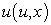to be in the form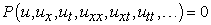(1)

Step 1. Seek solitary wave solutions of Eq. (3) by taking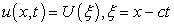and transform Eq. (3) to the ordinary differential equation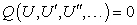(2)

where prime denotes the derivative with respect to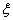.

Step 2. If possible, integrate Eq. (3) term by term one or more times. This yields constant(s) of integration. For simplicity, the integration constant(s) can be set to zero.

Step 3. According to He’s semi-inverse method, we construct the following trial-functional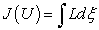(3)

where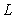is an unknown function of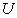and its derivatives.

There exist alternative approaches to the construction of the trial-functionals, see Refs [1, 2].

Step 4. By the Ritz method, we can obtain different forms of solitary wave solutions, in the form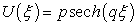(4)

where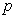and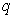are constants to be further determined.

Substituting Eq. (5) into Eq. (6) and making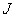stationary with respect toandresults in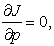(5)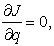(6)

Solving simultaneously Eqs. (5) and (6) we obtainand. Hence, the solitary wave solution (4) is well determined.

### 3. He’s Semi-inverse Method for (2 + 1)- Dimensional Boussinesq Equation

Consider the (2 + 1)-dimensional Boussinesq equation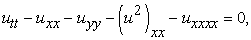(7)

Using the wave variable reduces it to an ODE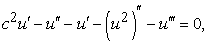(8)

Integrating twice and setting the constants of integration to zero, we obtain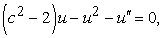(9)

According to Ref. , By He’s semi-inverse method , we can arrive at the following variational formulation: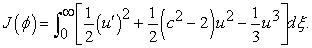(10)

We assume the soliton solution in the following form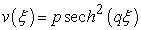(11)

where,is an unknown constant to be further determined.

By Substituting Eq. (11) into Eq. (10) we obtain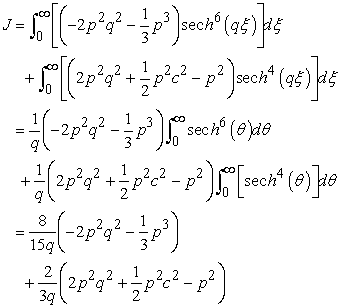(12)

For makingstationary with respect toandresults in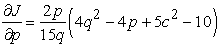(13-1)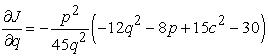(13-2)

or simplifying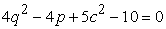(14-1)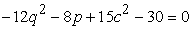(14-2)

From Eqs. (14-1) and (14-2), we can easily obtain the following relations: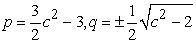(15)

So the solitary wave solution can be approximated as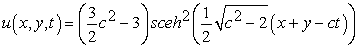(16)

In this solution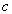is an arbitrary complex parameter.( For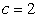, see Figure 1)

### 4. He’s Semi-inverse Method for (2 + 1)-Dimensional Breaking Soliton Equation

We now consider the (2 + 1)-dimensional breaking soliton equations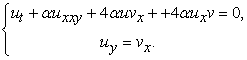(17)

Using the wave variable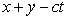reduces it to an ODE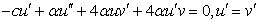(18)

Integrating the second equation in the system and neglecting constants of integration we find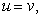(19)

Substituting (32) into the first equation of the system and integrating we find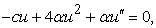(20)

According to Ref. , By He’s semi-inverse method , we can arrive at the following variational formulation: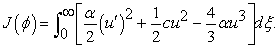(21)

We assume the soliton solution in the following form(22)

where,is an unknown constant to be further determined.

By Substituting Eq. (22) into Eq. (21) we obtain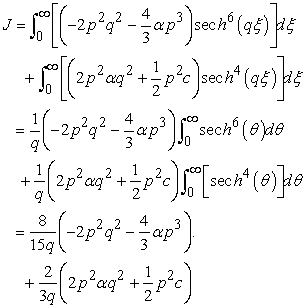(23)

For makingstationary with respect toandresults in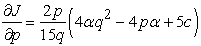(24-1)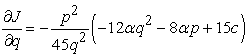(24-2)

or simplifying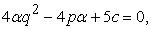(25-1)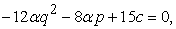(25-2)

From Eqs. (25-1) and (25-2), we can easily obtain the following relations: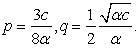(26)

So the solitary wave solution can be approximated as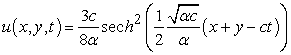(27)

And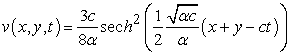(28)

In this solutionsis an arbitrary complex parameter.( For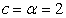, see Figure 2)

### 5. Conclusions

In this paper, by using the He’s semi-inverse method; we obtained some solitary solutions of (2+1)-dimensional Boussinesq and breaking soliton equations. He’s semi-inverse method is a very dominant instrument to find the solitary solutions for various nonlinear equations.

### References

  He, J. H., “Some asymptotic methods for strongly nonlinear equations”, Internat. J. Modern Phys. B, Vol. 20, 2006, 1141-1199.In article CrossRef  He, J. H., “Variational principles for some nonlinear partial differential equations with variable coefficients”, Chaos, Solitons and Fractals, Vol. 19, 2004, 847-851.In article CrossRef  Najafi, M., Arbabi, S., Najafi, M., “New application of sine-cosine method for the generalized (2+1)-dimensional nonlinear evolution equations”, International Journal of Advanced Mathematical Sciences, Vol. 1, No. 2, 2013, 45-49.In article